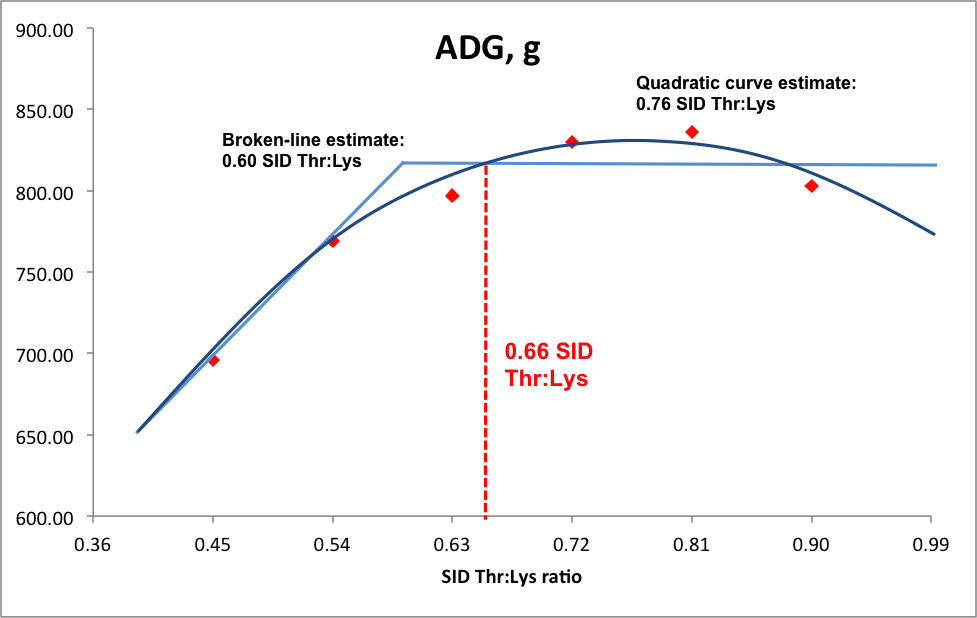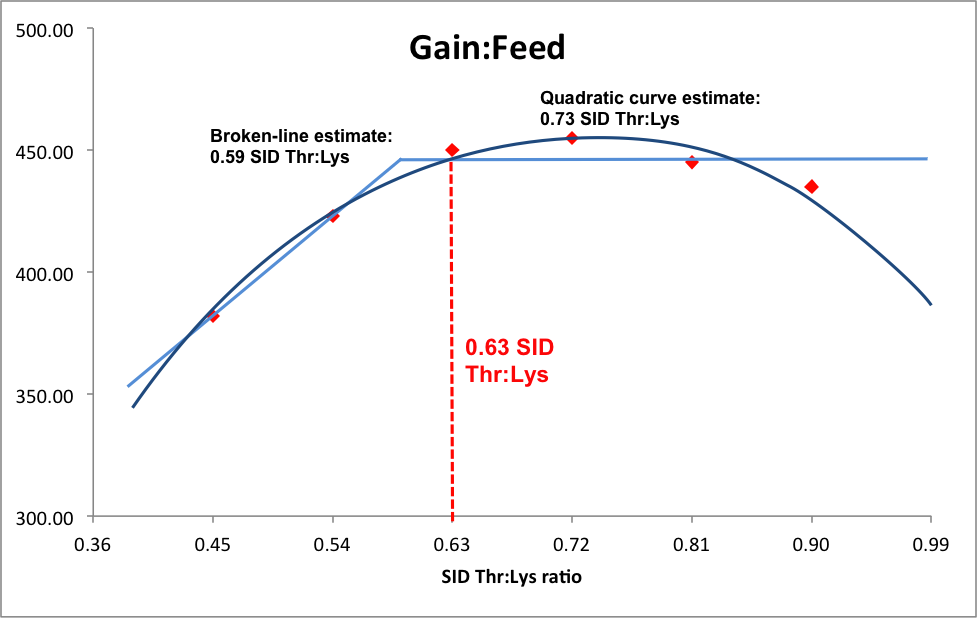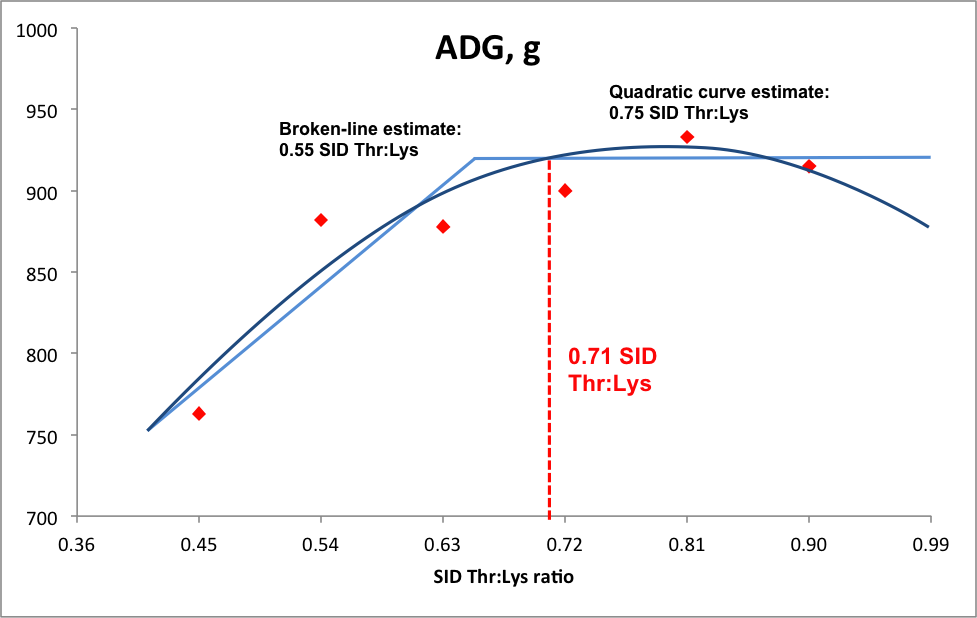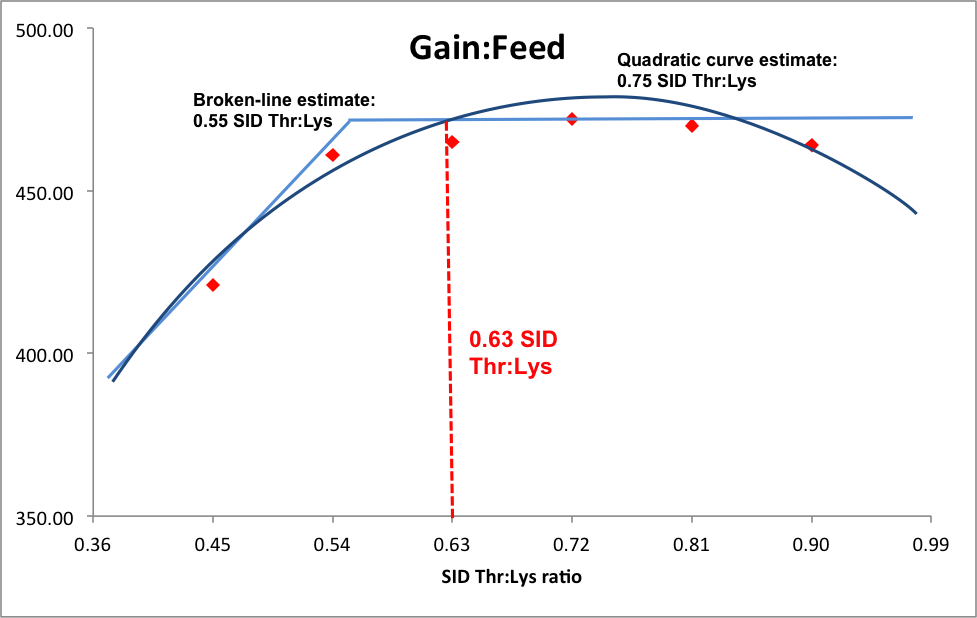# Effects of fiber on the optimal threonine:lysine ratio for 25 to 50 kg growing gilts

Use of high-fiber, low-cost ingredients, such as co-products from grain processing industries, in swine diets is increasing. Pigs fed diets containing high levels of fiber have increased intestinal mass due to increased amount of microbial fermentation in the hindgut. Therefore, they also have increased endogenous loss of amino acids in the form of mucins, the proteins that line the intestinal tract. The abrasiveness of fiber stimulates the secretion of mucins as well. These factors may cause the threonine requirement to be increased in high fiber diets, because threonine is present in large amounts in mucins. Therefore, an experiment was conducted to determine the effects of dietary fiber on the optimum threonine:lysine ratio (Thr:Lys) in 25 to 50 kg growing gilts.

## Materials and methods

A total of 192 gilts with average initial body weight of 26.29 kg were fed one of 12 diets. The low-fiber basal diet contained approximately 0.40% SID Thr and 0.90% SID Lys. The high-fiber basal diet was formulated by adding 15% soybean hulls to the low-fiber basal diet at the expense of corn starch. Ten additional diets were formulated by adding crystalline L-Thr to each basal diet to create diets containing SID Thr:Lys ratios of 0.45, 0.54, 0.63, 0.72, 0.81, and 0.90. Pigs were weighed at the beginning of the study and on the 28th day, and final body weight, average daily gain (ADG), average daily feed intake (ADFI), and gain:feed ratio (G:F) were calculated.

Broken-line and quadratic analysis were used for each of the growth performance measures. In broken-line analysis, determinations for requirements are defined as the intersection point between the slope and plateau portion of the model. Quadratic analysis fits a quadratic line to the data, and estimates the requirement as the level determined by the apex of the curve. Broken line analysis tends to yield conservative estimates, while quadratic analysis may overestimate requirements. Therefore, a combination of the two types of analyses was used. The concentration of Thr at which the quadratic regression line and the plateau value from the broken line analysis intersected was used to estimate the ideal SID Thr:Lys ratio for those growth performance parameters which differed both linearly and quadratically. The estimated ideals within each fiber level were averaged, and the average was determined to be the ideal SID Thr:Lys ratio.

## Growth performance

For pigs fed the low-fiber diets, ADG and G:F increased both linearly (P < 0.01) and quadratically (P < 0.05) as the concentration of Thr increased in the diets (Table 1). Final body weight increased linearly (P < 0.01) as Thr concentration increased. ADFI was not affected by the Thr concentration in the diets.

For pigs fed the high-fiber diets, ADG and G:F increased both linearly (P < 0.001) and quadratically (P < 0.05) as the concentration of Thr increased in the diets. Final BW and ADFI increased linearly (P < 0.05) as Thr concentration increased.

## Thr:Lys requirement estimates

For pigs fed the low-fiber diets, broken-line analyses estimated the optimum SID Thr:Lys ratio to be 0.60 for ADG (Figure 1) and 0.59 for G:F (Figure 2). Quadratic analyses estimated the optimum SID Thr:Lys ratio to be 0.76 for ADG (Figure 1) and 0.73 for G:F (Figure 2). Combining the broken-line and quadratic analyses yielded an estimated optimum SID Thr:Lys ratio of 0.66 for ADG (Figure 1) and 0.63 for G:F (Figure 2).

For pigs fed the high-fiber diets, broken-line analyses estimated the optimum SID Thr:Lys ratio to be 0.66 for ADG (Figure 3) and 0.55 for G:F (Figure 4). Quadratic analyses estimated the optimum SID Thr:Lys ratio to be 0.80 for ADG (Figure 3) and 0.75 for G:F (Figure 4). Combining the broken-line and quadratic analyses yielded an estimated optimum SID Thr:Lys ratio of 0.71 for ADG (Figure 3) and 0.63 for G:F (Figure 4).

## Key points

• For 25 to 50 kg growing gilts fed high-fiber diets, ADG will be optimized with a Thr:Lys ratio is 0.71.
• For 25 to 50 kg growing gilts fed low-fiber diets, the ideal Thr:Lys ratio to optimize ADG is 0.66.
• The ideal Thr:Lys ratio to optimize G:F in 25 to 50 kg growing gilts is 0.63, regardless of fiber content of the diets.

Table 1. Growth performance of pigs fed experimental diets

 Thr:Lys Contrasts (P-value) 0.45 0.54 0.63 0.72 0.81 0.9 SEM Linear Quadratic Low Fiber Initial BW, kg 26.16 26.68 26.68 25.73 26.73 26.39 0.8 0.963 0.969 ADG, g 696 769 797 830 836 803 28.9 <0.01 <0.05 ADFI, g 1,785 1,799 1,777 1,812 1,862 1,830 102 0.376 0.917 G:F 382 423 450 455 445 435 20.9 0.001 <0.001 Final BW, kg 45.66 49.43 49.56 49.5 50.13 50.36 1.15 <0.01 0.132 High Fiber Initial BW, kg 26.47 26.85 26.72 26.57 26.28 26.72 1.31 0.924 0.964 ADG, g 763 882 878 900 933 915 35.48 <0.001 <0.05 ADFI, g 1,828 1,872 1,835 1,864 1,945 1,989 64.9 <0.05 0.409 G:F 421 461 465 472 470 464 12.91 <0.01 <0.01 Final BW, kg 48.14 51.86 51.67 52.08 52.7 52.64 1.61 <0.05 0.145Figure 1. Fitted linear breakpoint and quadratic plots of ADG as a function of standardized ileal digestible Thr to Lys ratio with observed treatment means in pigs fed low fiber diets.Figure 2. Fitted linear breakpoint and quadratic plots of G:F as a function of standardized ileal digestible Thr to Lys ratio with observed treatment means in pigs fed low fiber diets.Figure 3. Fitted linear breakpoint and quadratic plots of ADG as a function of standardized ileal digestible Thr to Lys ratio with observed treatment means in pigs fed high fiber diets.Figure 4. Fitted linear breakpoint and quadratic plots of G:F as a function of standardized ileal digestible Thr to Lys ratio with observed treatment means in pigs fed high fiber diets.

Keywords:
Authors:
Publication Type: